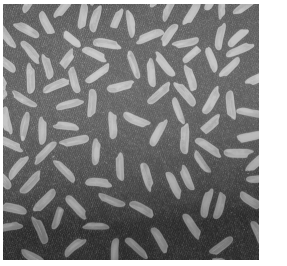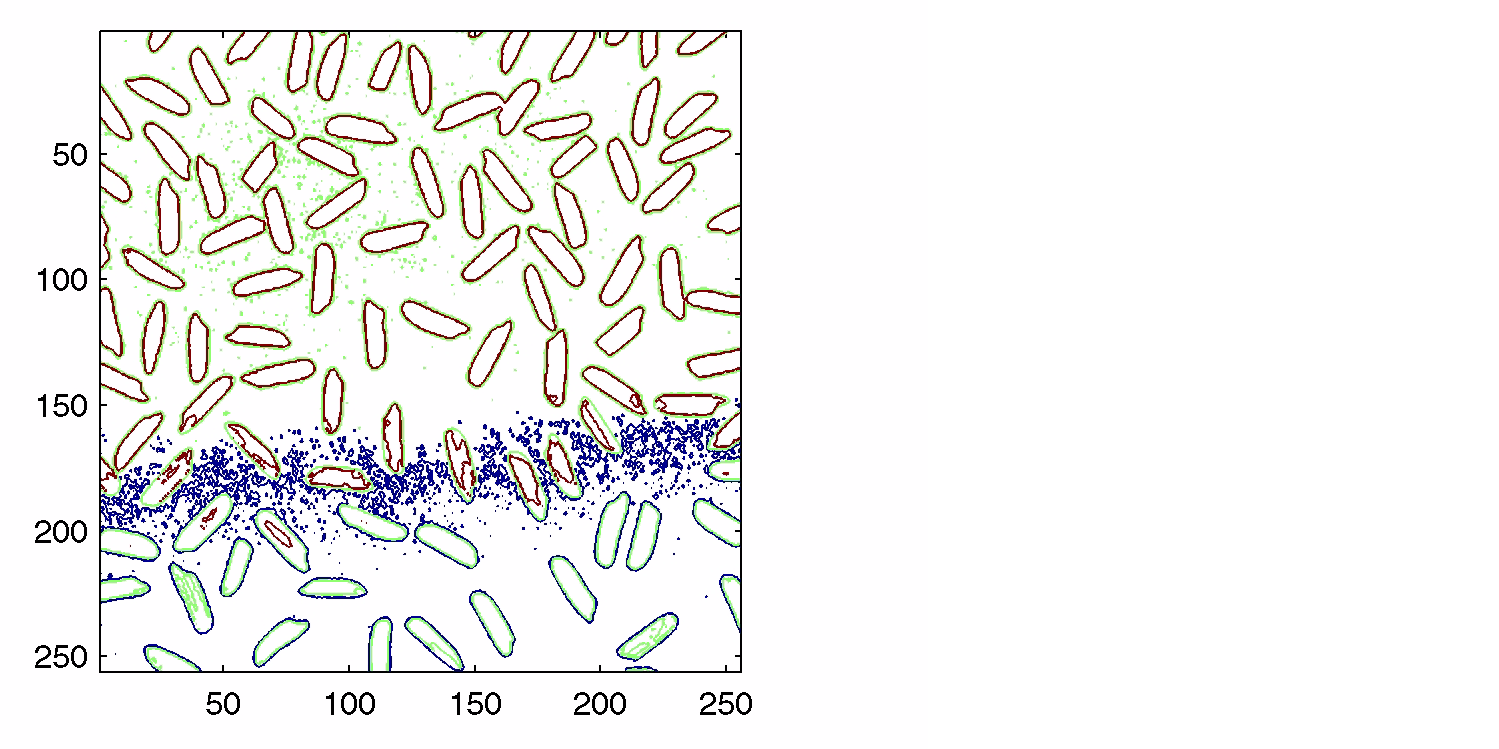Image Processing Toolbox User's GuideDisplaying a Contour Plot of Image Data

You can use the toolbox function `imcontour` to display a contour plot of the data in a grayscale image. A contour is a path in an image along which the image intensity values are equal to a constant. This function is similar to the `contour` function in MATLAB, but it automatically sets up the axes so their orientation and aspect ratio match the image.

This example displays a grayscale image of grains of rice and a contour plot of the image data:

1. Read a grayscale image and display it.
• ```I = imread('rice.png');
imshow(I)```
2. Display a contour plot of the grayscale image.
• ```figure, imcontour(I,3)```

You can use the `clabel` function to label the levels of the contours. See the description of `clabel` in the MATLAB Function Reference for details.Getting the Intensity Profile of an Image Creating an Image Histogram